Bihar Board 12th Maths Model Papers

## Bihar Board 12th Maths Model Question Paper 5 in English Medium

Section – I

Time : 3 Hours 15 Min
Full Marks: 100

Instructions for the candidates

1. Candidates are required to give their answers in their own words as far as practicable.
2. Figure in the right-hand margin indicates full marks.
3. While answering the questions, the candidate should adhere to the word limit as far as practicable.
4. 15 Minutes of extra time has been allotted for the candidate to read the questions carefully.
5. This question paper is divided into two sections. Section-A and Section-B.
6. In Section A, there are 1-50 objective type questions which are compulsory, each carrying 1 mark. Darken the circle with blue/black ball pen against the correct option on the OMR Sheet provided to you. Do not use Whitener/Liquid/ Blade/Nail on OMR Sheet otherwise result will be invalid.
7. In section-B, there are 25 short answer type questions (each carrying 2 marks), out of which only 15 (fifteen) questions are to be answered. A part from this there is 08 Long Answer Type questions (each carrying 5 marks), out of which 4 questions are to be answered.
8. Use of any electronic device is prohibited.

Objective Type Questions

There are 1 to 50 objective type questions with 4 options, choose the correct option which, is to be answered on OMR Sheet. (50 × 1 = 50)

Question 1.
If the function f : N → N defined as f(x) = 4x + 3, then f-1(x) = _________
(a) 4x – 3
(b) $$\frac{4 x-3}{2}$$
(c) $$\frac{x+3}{2}$$
(d) $$\frac{x-3}{4}$$
(d) $$\frac{x-3}{4}$$

Question 2.
The set natural numbers defined, as the relation less than is
(a) only reflexive
(b) only symmetric
(c) equivalence
(d) only transitive
(d) only transitiveQuestion 3.
The operation * defined as a* b such that a2 + b2 then (1*2) *5 is
(a) 3125
(b) 625
(c) 125
(d) 50
(d) 50

Question 4.
If the function f : R → R defined as f(x) = $$\left(3-x^{3}\right)^{1 / 3}$$ then fof(x) is
(a) $$x^{1 / 3}$$
(b) x3
(c) (3 – x3)
(d) x
(d) x

Question 5.
$$\sin ^{-1}\left(\sin \frac{2 \pi}{3}\right)=$$ = _______
(a) $$\frac{2 \pi}{3}$$
(b) $$\frac{\pi}{6}$$
(c) $$\frac{4 \pi}{3}$$
(d) $$\frac{\pi}{3}$$
(d) $$\frac{\pi}{3}$$Question 6.
tan-1 √3 – cot-1(-√3) = _______
(a) π
(b) 0
(c) 2√3
(d) $$-\frac{\pi}{2}$$
(d) $$-\frac{\pi}{2}$$

Question 7.
$$\cos ^{-1}\left(\cos \frac{7 \pi}{6}\right)$$ is equal to
(a) $$\frac{7 \pi}{6}$$
(b) $$\frac{5 \pi}{6}$$
(c) $$\frac{\pi}{3}$$
(d) $$\frac{\pi}{6}$$
(b) $$\frac{5 \pi}{6}$$

Question 8.
A matrix A = [aij]n×n is symmetric if
(a) aij = 0
(b) aij = -aji
(c) aij = aji
(d) aij = 1
(c) aij = aji

Question 9.
$$\int { \frac { { sec }^{ 2 }x }{ { cosec }^{ 2 }x } } dx\quad =$$ ___________
(a) x – tan x + c
(b) tan x + x + c
(c) tan x – x + c
(d) -tan x – x + c
(c) tan x – x + cQuestion 10.
$$\int \frac{\cos 2 x}{(\sin x+\cos x)^{2}} d x=$$ _______
(a) $$\frac{1}{\sin x+\cos x}+c$$
(b) log (sinx + cosx) + c
(c) log |sinx – cosx| + c
(d) $$\frac{1}{(\sin x+\cos x)^{2}}+c$$
(b) log (sinx + cosx) + c

Question 11.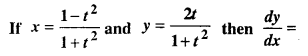q11
(a) $$\frac{-y}{x}$$
(b) $$\frac{y}{x}$$
(c) $$\frac{-x}{y}$$
(d) $$\frac{x}{y}$$
(a) $$\frac{-y}{x}$$

Question 12.
If √x + √y = 5 then $$\frac{d y}{d x}$$ = ? at (4, 9).
(a) $$\frac{2}{3}$$
(b) $$\frac{3}{2}$$
(c) $$\frac{-3}{2}$$
(d) $$\frac{-3}{3}$$
(b) $$\frac{3}{2}$$

Question 13.
$$\int \tan ^{2} \frac{x}{2} d x=$$ __________
(a) $$\tan \frac{x}{2}-x+c$$
(b) $$\tan \frac{x}{2}+x+c$$
(c) $$2 \tan \frac{x}{2}+x+c$$
(d) $$2 \tan \frac{x}{2}-x+c$$
(a) $$\tan \frac{x}{2}-x+c$$

Question 14.qim 14
(a) $$\frac{\pi}{4}$$
(b) $$-\frac{\pi}{4}$$
(c) 0
(d) $$\frac{\pi}{2}$$
(a) $$\frac{\pi}{4}$$

Question 15.
Let A be a square matrix of order 3 × 3 then |KA| is equal to.
(a) K|A|
(b) K2 |A|
(c) K3 |A|
(d) 3K|A|
(c) K3 |A|Question 16.
If $$A=\left|\begin{array}{lll} a_{11} & a_{12} & a_{13} \\ a_{21} & a_{22} & a_{23} \\ a_{31} & a_{33} & a_{33} \end{array}\right|$$ and cofactor of aij is Aij then which of following is the value of Δ
(a) a11 A31 + a12 A32 + a13 A33
(b) a11 A11 + a12 A21 + A13 A31
(c) a21 A11 + a22 A22 + a23 A13
(d) a11 A11 + a21 A21 + a31 A31
(d) a11 A11 + a21 A21 + a31 A31

Question 17.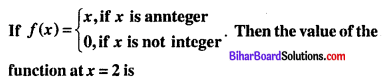(a) Continuous
(b) Discontinuous
(c) Well defined
(d) None of these
(b) Discontinuous

Question 18.
If y = log (log x) then the value of $$\frac{d y}{d x}$$ is:
(a) x log x
(b) $$\frac{1}{x \log x}$$
(c) $$\frac{1}{\log x}$$
(d) $$\frac{1}{x}$$
(b) $$\frac{1}{x \log x}$$

Question 19.
If y = sec (tan-1 x) Then $$\frac{d y}{d x}$$ is equl to
(a) $$\frac{x}{\sqrt{1+x^{2}}}$$
(b) $$\frac{-x}{\sqrt{1+x^{2}}}$$
(c) $$\frac{x}{\sqrt{1-x^{2}}}$$
(d) None of these
(a) $$\frac{x}{\sqrt{1+x^{2}}}$$

Question 20.
If f(x) = sin(x2) Then $$\frac{d f}{d x}$$ is equal to
(a) 2x sin x2
(b) 2x cos x2
(c) 2cos x
(d) 2x sin x
(b) 2x cos x2Question 21.
The rate of change of the area of the circle with respect to r = 7 cm is
(a) 42 cm2/cm
(b) 44 cm2/cm
(c) 28 cm2/cm
(d) 22 cm2/cm
(b) 44 cm2/cm

Question 22.
The function f(x) = x3 – 6x2 + 12x – 18 is:
(a) Strictly decreases for each x ∈ R
(b) Strictly increasing ∀ x ∈ R
(c) Increasing in ] -∞, 0 [ and decreasing in [0, ∞[
(d) None of these
(b) Strictly increasing ∀ x ∈ R

Question 23.
The total revenue in Rupees received from the sale of x units of a product is given by R (x) = 3x2 + 36x + 5. The marginal revenue, when x = 15 is:
(a) Rs. 116
(b) Rs. 96
(c) Rs. 90
(d) Rs. 126
(d) Rs. 126

Question 24.
What is the slope of the tangent to the curve y = x3 – x + 1 at the point whose x-coordinate is 2?
(a) 11
(b) 21
(c) 31
(d) 41
(a) 11Question 25.
$$\int \frac{10 x^{9}+10^{x} \log e^{10}}{x^{10}+10^{x}}$$ is equal to
(a) 10x – x10 + C
(b) 10x + x10 + C
(c) (10x – x10)-1 + C
(d) log (10x + x10) + C
(d) log (10x + x10) + C

Question 26.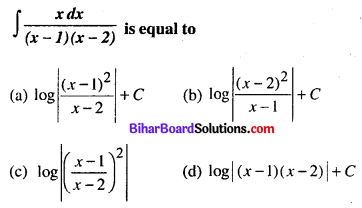(b) $$\log \left|\frac{(x-2)^{2}}{x-1}\right|+C$$

Question 27.(a) 2
(b) $$\frac{3}{4}$$
(c) 0
(d) -2
(c) 0

Question 28.
If f(a + b – x) = f(x) then $$\int_{a}^{b} x f(x) d x$$ is equal to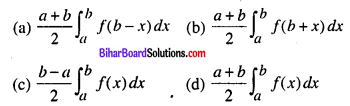(d) $$\frac{a+b}{2} \int_{a}^{b} f(x) d x$$

Question 29.
Area lying in the first quadrant and bounded by the circle x2 + y2 = 4 and the lines x = 0, and x = 2 is:
(a) π
(b) $$\frac{\pi}{2}$$
(c) $$\frac{\pi}{3}$$
(d) $$\frac{\pi}{4}$$
(a) π

Question 30.
The value of $$\int \frac{\sin ^{2} x-\cos ^{2} x}{\sin ^{2} x \cos ^{2} x} d x$$ is equal to
(a) tan x + cot x + C
(b) tan x + cosec x + C
(c) -tan x + cot x + C
(d) tan x + sec x + C
(a) tan x + cot x + C

Question 31.
The value of $$\int_{0}^{\pi / 2} \cos 2 x d x$$ is:
(a) 0
(b) 1
(c) 2
(d) 3
(a) 0

Question 32.
The degree of diff. eqn.(a) 3
(b) 2
(c) 1
(d) None of these
(d) None of these

Question 33.
The degree and order of the differential equation(a) 4, 2
(b) 2, 4
(c) 3, 4
(d) 4, 3
(b) 2, 4

Question 34.
The general solution of diff. eqn $$\frac{y d x-x d y}{y}=0$$ is:
(a) xy = c
(b) x = cy2
(c) y = cx
(d) y = cx2
(c) y = cx

Question 35.
The number of arbitrary constant in the solution of diff. eqn of fourth-order is
(a) 0
(b) 2
(c) 3
(d) 4
(d) 4Question 36.
The general solution of diff. eqn $$\frac{d y}{d x}=e^{x+y}$$ is
(a) ex + ey = c
(b) ex + e-y = c
(c) e-x + ey = c
(d) e-x + e-y = c
(b) ex + e-y = c

Question 37.
Let the vectors $$\vec{a}$$ and $$\vec{b}$$ be such that $$|\vec{a}|=3$$ and $$|\vec{b}|=\frac{\sqrt{2}}{3}$$, then $$\vec{a} \times \vec{b}$$ is a unit vector if the angle between $$\vec{a}$$ and $$\vec{b}$$ is
(a) $$\frac{\pi}{6}$$
(b) $$\frac{\pi}{4}$$
(c) $$\frac{\pi}{3}$$
(d) $$\frac{\pi}{2}$$
(b) $$\frac{\pi}{4}$$

Question 38.
If $$\vec{a}=2 \hat{j}-5 \hat{j}+\hat{k}$$ and $$\vec{b}=\hat{i}+2 \hat{j}-\hat{k}$$ Then
(a) $$\vec{a} \cdot \vec{b}=0$$
(b) $$\vec{a} \cdot \vec{b} \neq 0$$
(c) $$\vec{a} \cdot \vec{b}=-9$$
(d) $$\vec{a}+\vec{b}$$
(c) $$\vec{a} \cdot \vec{b}=-9$$

Question 39.
l2 + m2 + n2 is equal to
(a) 0
(b) 1
(c) -1
(d) None of these
(b) 1

Question 40.
The Dr’s of any line is 2, -1, -2 then dc’s is: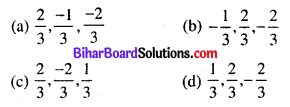q40
(a) $$\frac{1}{3}, \frac{2}{3},-\frac{2}{3}$$

Question 41.
The direction ratios of the line $$\frac{x-5}{3}=\frac{y+4}{7}=\frac{z-6}{2}$$ is:
(a) 3, 2, 7
(b) 3, 7, 2
(c) 2, 3, 7
(d) 7, 3, 2
(b) 3, 7, 2

Question 42.
The lines with dr’s a1, b1, c1 and a2, b2, c2 are parallel if
(a) a1a2 – b1b2 – c1c2 = 0
(b) a1a2 + b1b2 + c1c2 = 0
(c) a1b1 + a2b2 + a1b2 = 0
(d) $$\frac{a_{1}}{a_{2}}=\frac{b_{1}}{b_{2}}=\frac{c_{1}}{c_{2}}$$
(d) $$\frac{a_{1}}{a_{2}}=\frac{b_{1}}{b_{2}}=\frac{c_{1}}{c_{2}}$$

Question 43.
The angle between the two lines with direction cosines are l1, m1, n1 and l2, m2, n2 is θ. Then θ is equal to
(a) l1l2 + m1m2 + n1n2
(b) l1l2 – m1m2 – n1n2
(c) l1m1n1 + l2m2n2
(d) None of these
(a) l1l2 + m1m2 + n1n2

Question 44.
Which of the following equations correponds to inequations ax + by ≥ c, ax + by > c, ax + by ≤ c and ax + by < c is:
(a) ax + by = c
(b) ax + by = 0
(c) bx + ay = c
(d) None of these
(a) ax + by = cQuestion 45.
Which of the following is non-negative constraints
(a) x ≤ 0, y ≤ 0
(b) x ≤ 0, y ≥ 0
(c) x ≥ 0, y ≥ 0
(d) None of these
(c) x ≥ 0, y ≥ 0

Question 46.
If P(A) = $$\frac{3}{8}$$, P(B) = $$\frac{1}{2}$$, P(A∩B) = $$\frac{1}{4}$$, then P(A/B) = _______
(a) $$\frac{1}{4}$$
(b) $$\frac{1}{2}$$
(c) $$\frac{2}{3}$$
(d) $$\frac{3}{8}$$
(b) $$\frac{1}{2}$$

Question 47.
If A and B are two independent events then
(a) P(AB’) = P(A) P(B)
(b) P(AB’) = P(A) P(B’)
(c) P(AB’) = P(A’) + P(B)
(d) P(AB’) = P(A) + P(B’)
(b) P(AB’) = P(A) P(B’)

Question 48.
If A and B are two envents such that P (A) = $$\frac{1}{3}$$, P(B) = $$\frac{1}{4}$$, P (A∩B) = $$\frac{1}{5}$$ thenP(A/B) is:
(a) $$\frac{1}{5}$$
(b) $$\frac{2}{5}$$
(c) $$\frac{3}{5}$$
(d) $$\frac{4}{5}$$
(d) $$\frac{4}{5}$$

Question 49.
If P(A) = $$\frac{3}{8}$$, P(B) = $$\frac{5}{8}$$ and P(A∪B) = $$\frac{3}{4}$$, then P(B/A) is:
(a) $$\frac{1}{4}$$
(b) $$\frac{1}{3}$$
(c) $$\frac{2}{3}$$
(d) $$\frac{1}{2}$$
(c) $$\frac{2}{3}$$Question 50.
If A and B are two events such that P(A) + P(B) – P(A and B) = P(A) then
(a) $$P\left(\frac{B}{A}\right)=1$$
(b) $$P\left(\frac{B}{A}\right)=0$$
(c) $$P\left(\frac{A}{B}\right)=1$$
(d) $$P\left(\frac{A}{B}\right)=0$$
(c) $$P\left(\frac{A}{B}\right)=1$$

Section – II

Time : 2 Hours 5 Minutes
Total Marks: 50

Non-Objective Type Questions

Q. No. 1 to 25 are of Short Answer type carry of 2 marks each. Answer any 15 questions. (15 × 2 = 30)

Question 1.
If $$A=\left[\begin{array}{rr} -1 & 2 \\ 3 & 4 \end{array}\right]$$ and $$B=\left[\begin{array}{ll} 3 & -2 \\ 1 & 4 \end{array}\right]$$ suchthat 2A + B + X = 0, then find X.
Solution:
Given, 2A + B + X = 0 ⇒ X = -(2A + B)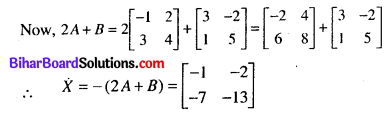Question 2.
Consider f : R → R given by f(x) = 4x + 3. show that f is invertible. Find the inverse of f also.
Solution:
Given f: R → R and f(x) = 4x + 3
f(x1) = f(x2)
or, 4x1 + 3 = 4x2 + 3
or, x1 = x2
f is one-one
Again, let y = 4x + 3
$$x=\frac{y-3}{4}$$
Each element in co-domain of y is a image of any one element in domain.
f is onto
since f is one-one and onto then f is invertible
Hence $$f^{-1}(x)=g(y)=\frac{y-3}{4}$$

Question 3.
Prove thatSolution: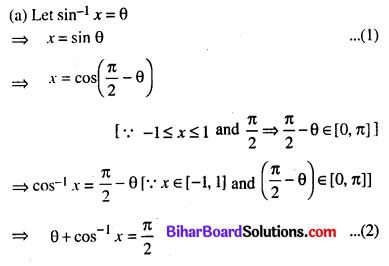Question 4.
For what value of x is:
$$\left[\begin{array}{lllll} 1 & 2 & 1 \end{array}\right]\left[\begin{array}{lll} 1 & 2 & 0 \\ 2 & 0 & 1 \\ 1 & 0 & 2 \end{array}\right]\left[\begin{array}{l} 0 \\ 2 \\ x \end{array}\right]=0$$Question 5.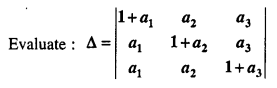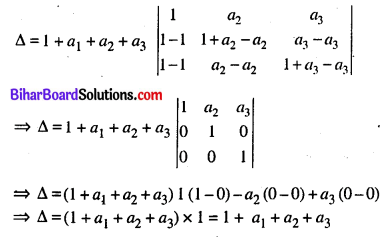Question 6.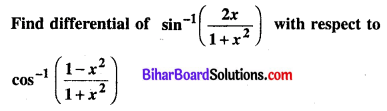Question 7.Question 8.
If x = a(θ + sinθ), y = a(1 – cosθ), then find $$\frac{d y}{d x}$$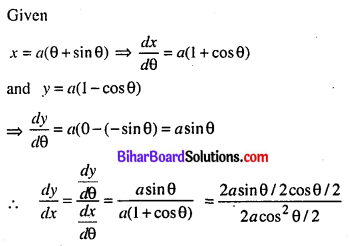Question 9.
Find the point at which the tangent to the curve $$y=\sqrt{4 x-3}-1$$ has its slope $$\frac{2}{3}$$
Solution:
Given curve $$y=\sqrt{4 x-3}-1$$
Differentiating both sides w.r.t. x, we get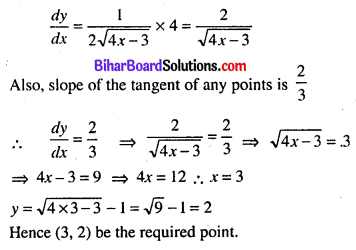Question 10.
If A = tan-1 2, and B = tan-1 3 then prove that C = $$\frac{\pi}{4}$$
Solution:
Let A = tan-1 2 = tan A = 2 and B = tan-1 3 ⇒ tan B = 3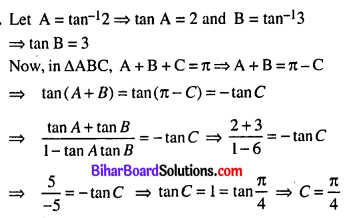Question 11.
Using differential, find the approximate value of √25.3 up to three places of decimal.
Solution:
Let y = √xQuestion 12.
Find the integral $$\frac{e^{\tan -1} x}{1+x^{2}}$$
Solution:Question 13.
Integrate: $$\int \tan ^{4} x d x$$
Solution:Question 14.
Evaluate: $$\int_{0}^{1} \frac{e^{x}}{1+e^{x}} d x$$
Solution: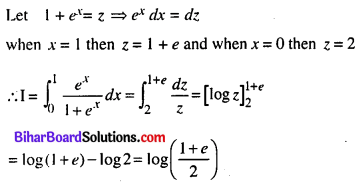Question 15.
Find the area enclosed by the circle x2 + y2 = a2
Solution:
x2 + y2 = a2
⇒ y2 = a2 – x2
⇒ y = $$\sqrt{a^{2}-x^{2}}$$
Area enclosed by the circle = 4 × (Given curve, A-axis and ordinates x = 0, x = a)Hence the area of a circle is πa2 sq. units

Question 16.
Solve: y dx – x dy = xy dx
Solution:
y dx – x dy = xy dx
⇒ x dy = y (1 – x) dxQuestion 17.
Solve: $$\frac{x d y}{d x}-y=x^{2}$$
Solution:Question 18.
Find the value of λ, which the planes $$\vec{r} \cdot(\hat{i}+2 \hat{j}+3 \hat{k})=13$$ and $$\vec{r} \cdot(\lambda \hat{i}+2 \hat{j}-7 \hat{k})=9$$ perpendiular to each other.
Solution:Question 19.
Find the area of the parallelogram whose adjacent sides are $$\overrightarrow{\mathrm{a}}=\hat{\mathrm{i}}+2 \hat{\mathrm{j}}+3 \hat{\mathrm{k}}$$ and $$\overrightarrow{\mathbf{b}}=3 \hat{\mathbf{i}}-2 \hat{\mathbf{j}}+\hat{\mathbf{k}}$$
Solution:Question 20.
Find the internally intercepts of the plane 2x + y – z = 5
Solution:
Given plane 2x + y – z = 5Hence the intercepts of the plane are $$\frac{5}{2}$$, 5 and -5 respectively

Question 21.
Find the shortest distance between the linesSolution:
Let PQ be the distance between the lines AB and CD.
If direction cosines of PQ is l, m, n. then 3l – m + n = 0 and -3l + 2m + 4n,
Now, 3l – m + n = 0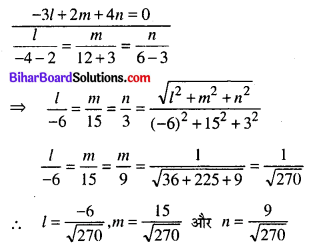Again, the coordinates of the point R and S on. the lines AB and CD are R(3, 8, -3) and (-3, -7, 6)
Now, PQ = Projection of RSQuestion 22.
The probabilities of solving a problem by three students are $$\frac{1}{2}, \frac{1}{3}, \frac{1}{4}$$; find the probability that the problem will be solved.
Solution:
Let A, B and C are the events for solving the problem by 1st, 2nd and 3rd students.Question 23.Solution: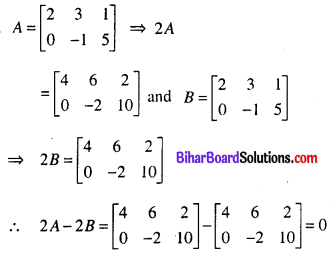Question 24.
Find the value of x, y and z if $$\left[\begin{array}{cc} x+y & 2 \\ 5+z & x y \end{array}\right]=\left[\begin{array}{ll} 6 & 2 \\ 5 & 8 \end{array}\right]$$
Solution:
Given, $$\left[\begin{array}{cc} x+y & 2 \\ 5+z & x y \end{array}\right]=\left[\begin{array}{ll} 6 & 2 \\ 5 & 8 \end{array}\right]$$
x + y = 6, 5 + z = 5, xy = 8
⇒ y = 6 – x
⇒ xy = x(6 – x) = 8
⇒ 6x – x2 = 8
⇒ x2 – 6x + 8 = 0
⇒ x2 – 4x – 2x + 8 = 0
⇒ (x – 4) (x – 2) = 0
⇒ x – 4 = 0 or x – 2 = 0
⇒ x = 2, 4
y = 4, 2 and z = 0
Thus x = 2, 4, y = 4, 2, and z = 0

Question 25.
Prove that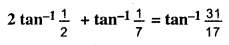Solution:Questions No. 26 to 33 are Long Answer Type Questions. Each question carries 5 marks. Answer any 4 questions. (4 × 5 = 20)

Question 26.
A rectangular sheet of 45 cm by 24 cm is to be made into a box without top, by cutting off square from each corner and folding up the flaps to form the box. What should be the side of the square to be cut off so that the volume of the box is maximum.
Solution:
Let each side of the square cut-off from each comer be x cm.
Sides of the rectangular box are (45 – 2x), (24 – 2x) and x cm.
Then volume of the box.
V = (45 – 2x) (24 – 2x) (x) = 2x (45 – 2x) (12 – x) = 2 (2x3 – 69x2 + 540x)
$$\frac{d V}{d x}$$ = 2(6x2 – 138x + 540) = 12(x2 – 23x + 90)
For max. and min. value. $$\frac{d V}{d x}$$ = 0
or 12(x2 – 23x + 90) = 0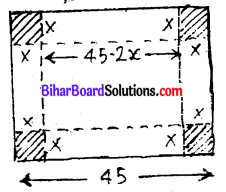or, (x – 5) (x – 18) = 0
But x is not greater than 12.
x = 5
$$\frac{d^{2} V}{d x^{2}}$$ = 12(2x – 23) = 12(10 – 23) = -156 = -ve [∵ x = 5]
Hence V is maximum at x = 5 i.e. square of sides 5 cm is cut off from.

Question 27.
Find the absolute maximum and minimum values of functions f given by f(x) = 2x3 – 15x2 + 36x + 1 at [1, 5]
Solution:
f(x) = 2x3 – 5x2 + 36x + 1
⇒ f'(x) = 6x2 – 30x + 36
⇒ f(x) = 6(x2 – 5x + 6) = 6(x – 3)(x – 2)
If f'(x) = 0 then x = 2, x = 3
Now, evaluate the value off at interval [1, 5] i.e. x = 1, x = 2 and x = 3
f(x) = 2x3 – 15x2 + 36x + 1
⇒ f(1) = 2 – 15 + 36 + 1 = 39 – 15 = 24
⇒ f(2) = 2 × 23 – 15 × 22 + 36 × 2 + 1 = 16 – 60 + 72 + 1 = 80 – 60 = 29
⇒ f(3) = 2 × 33 – 15 × 32 + 36 × 3 + 1 = 54 – 135 + 108 + 1 = 163 – 135 = 28
⇒ f(5) = 2 × 53 – 15 × 52 + 36 × 5 + 1 = 250 – 375 + 180 + 1 = 56
Thus the absolute maximum value of f on [1, 5] is 56 at x = 5 and minimum is 24 at x = 1Question 28.
Find the area of the curve bounded by x2 = 4y and the line x = 4y – 2.
Solution:
Given curve x2 = 4y …(1 )
Which is an upward parabola with vertex (0, 0) and is symmetrical about y-axis and equation of the line.
x = 4y – 2 …(2)
Solving (1) and (2), we get,
(4y – 2)2 = 4y
⇒ 16y2 – 16y + 4 = 4y
⇒ 16y2 – 20y + 4 = 0
⇒ 4y2 – 5y + 1 = 0
⇒ (4y – 1) (y – 1) = 0
⇒ y = $$\frac{1}{4}$$, 1
From(2), when y = $$\frac{1}{4}$$, then x = 1 – 2 = -1
and when y = 1, then x = 4 – 2 = 2
The intersection of (1) and (2) is A(-1, $$\frac{1}{4}$$) and B (2, 1)
Clear from figure, shaded, region. OBDA be the required area.
Required Area = Area of region LOMBDA – Area of region LMBOA …(3)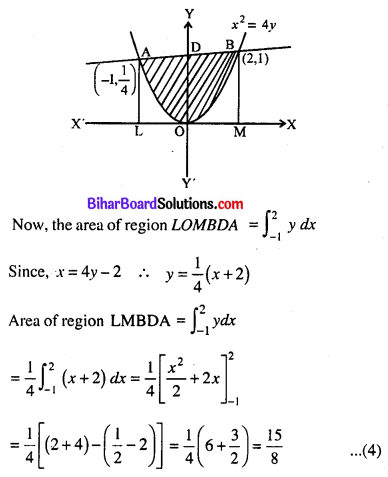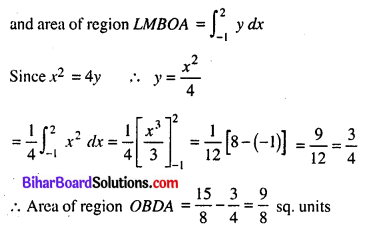Question 29.
Evaluate: $$\int_{0}^{\pi / 2}(\sqrt{\tan x}+\sqrt{\cot x}) d x$$
Solution:Question 30.
The two adjacent sides of parallelogram are $$\mathbf{2} \hat{\mathbf{i}}-\mathbf{4} \hat{\mathbf{j}}+\mathbf{5} \hat{\mathbf{k}}$$ and $$\hat{\mathbf{i}}-\mathbf{2} \hat{\mathbf{j}}-\mathbf{3} \hat{\mathbf{k}}$$. Find the unit vector parallel to its diagonal. Also find its area.
Solution:
Let the adjacent sides of a prallelogram are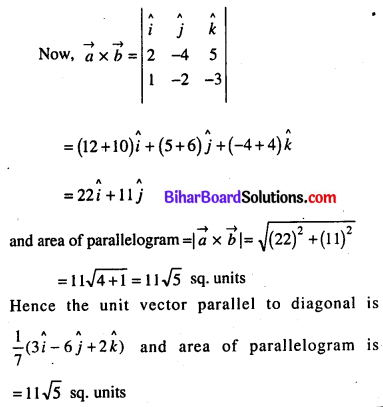Question 31.
Find the image of the point (1, 6, 3) in the line $$\frac{x}{1}=\frac{y-1}{2}=\frac{z-2}{3}$$
Solution:
Let P (1, 6, 3) be any point and AB be the given line.
Draw perpendicular PM from P to AB such that PM = P’M, where P’ be the image of P.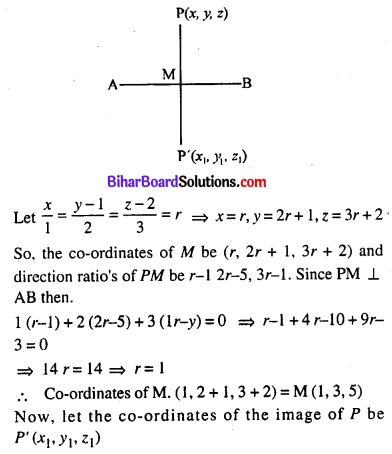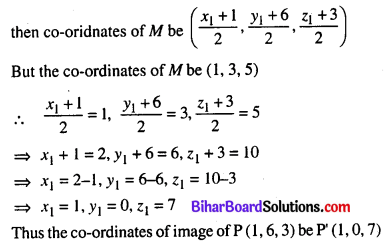Question 32.
Minimize Z = 200x + 500y subject to constraints; x + 2y ≥ 10, 3x + 4y ≤ 24, x ≥ 0, y ≥ 0.
Solution:
Minimize Z = 200x + 500y
x + 2y ≥ 10 ⇒ x + 2y = 10 ……. (1)
3x + 4y ≤ 24 ⇒ 3x + 4y = 24 …….. (2)
x ≥ 0, y ≥ 0 ⇒ x = 0, y = 0 ……… (3)
First of all draw the graph of the equations (1) to (3) corrsponds to inequations.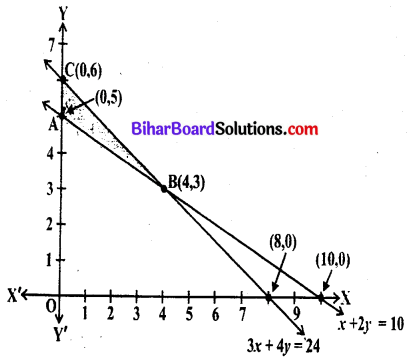On joining the points (10, 0), (0, 5) and (8, 0), (0, 6) we get two mutually lines which intersects at the point (4, 3). It is clear from figure shaded region ABC is feasible and bounded. The coordinates of the feasible region ABC are A (4, 3), B(0, 6) and C(0, 5)
Lastly, applying corner point method to find, the minimum value of objective function as follows.
Corner point – Z = 200x + 500y
(0, 5) ⇒ Z = 0 + 5000 × 5 = 2500
(4, 3) ⇒ Z = 200 × 4 + 500 × 3 = 2300
(0, 6) ⇒ Z = 200 × 0 + 500 × 6 = 3000
We see from the above table that the minimum value of Z is 2300 at the point (4, 3).
Thus minimum value of Z is 2300.

Question 33.
Solve the following LPP problem graphically: Maximize Z = 4x + y subject to constraints: x + y ≤ 50, 3x + y ≤ 90, x ≥ 0, y ≥ 0.
Solution:
Maximize Z = 4x + y
Subject to constraints: x + y ≤ 50 ⇒ x + y = 50 …… (1)
3x + y ≤ 90 ⇒ 3x + y = 90 …… (2)
x ≥ 0, y ≥ 0 ⇒ x = 0, y = 0 ….. (3)
First of all draw the graph of the equations (1) to (3) corresponds to inequations. It is clear from the figure shaded region OABC. be the feasible region and is bounded. The co-ordinate of the point B obtain by solving equation (1) and (2).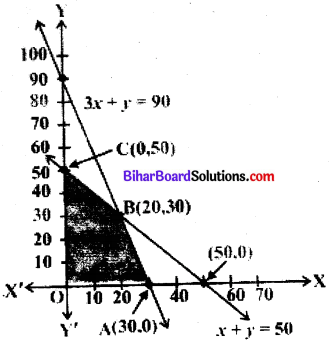It is clear from figure the co-ordinates of the comer points of the feasible region OABC are O(0, 0), A (30, 0), B (20, 30) and C(0, 50).
Lastly applying corner point method to find the maximum value of Z as follows:
Corner point – z = 4x + y
O(0, 5) ⇒ z = 4 × 0 + y
A(30, 3) ⇒ z = 4 × 0 + 0 = 0
B(20, 30) ⇒ z = 4 × 30 + 0 = 120
C(0, 50) ⇒ z = 4 × 20 + 30 = 110
We see from table that the maximum value of Z is 120 at the point A(30, 0).
Thus maximum value of Z is 120.